# HBSE 7th Class Maths Solutions Chapter 15 Visualising Solid Shapes Ex 15.1

Haryana State Board HBSE 7th Class Maths Solutions Chapter 15 Visualising Solid Shapes Ex 15.1 Textbook Exercise Questions and Answers.

## Haryana Board 7th Class Maths Solutions Chapter 15 Visualising Solid Shapes Exercise 15.1

Question 1.
Identify the nets which can be used to make cubes (cut out copies of the nets and try it) :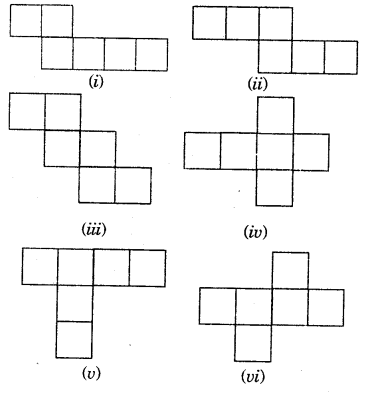Solution: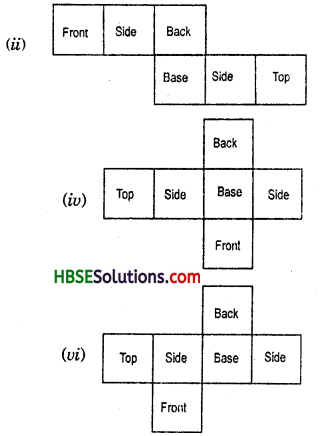Question 2.
Dice are cubes with dots on each face.
Opposite faces of a die always total to seven dots on them. Fig.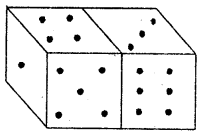Here are two nets to make dice (cubes); the numbers inserted in each square indicate the number of dots in that box.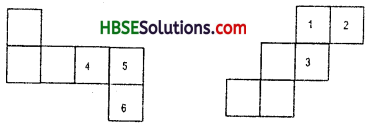Insert suitable numbers in the blanks, remembering that the number on the opposite faces should total to 7.
Solution: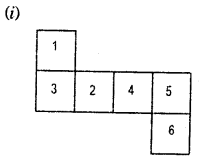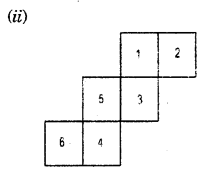Question 3.
Can this be a net for a die ? Explain your answer. (Shown Fig. 15.11.)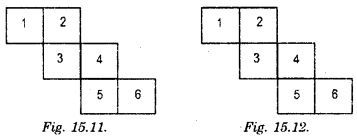Solution:
(Shown in Fig. 15.12). No, the net is not for die, because the sum of the opposite laces is not 7.Question 4.
Here is an incomplete net for making a cube. Complete it in at least two different ways. Remember that a cube has six faces. How many are there in the net here ? (Give two separate diagrams. If you like, you may use a squared sheet for easy manipulation.)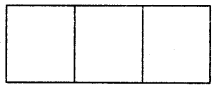Solution: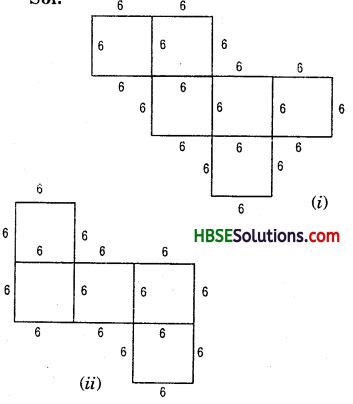Question 5.
Match the nets with appropriate solids :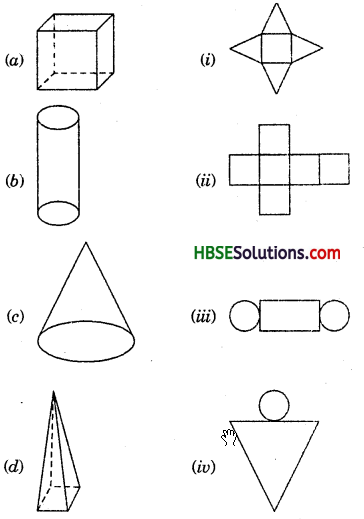Solution:
(a) → (ii)
(b) → (iii)
(c) → (iv)
(d) → (i)